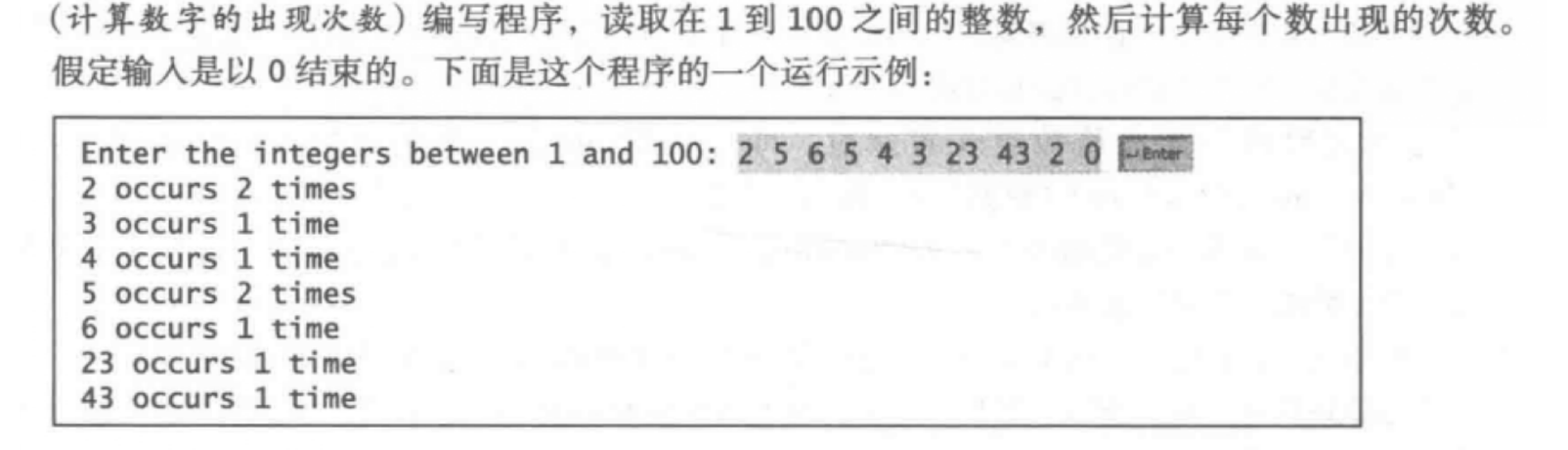• 直接将（变量名+双引号）赋给字符串； int a=100； string str=a+"
直接将（变量名+双引号）赋给字符串；
int a=100；
string str=a+""

展开全文• java 整数转化成字符串Given an integer and we have to convert it into string. 给定一个整数，我们必须将其转换为字符串Java conversion from Integer to String Java整数字符串的转换 To convert an ...

java 整数转化成字符串
Given an integer and we have to convert it into string.
给定一个整数，我们必须将其转换为字符串。
Java conversion from Integer to String
Java从整数到字符串的转换
To convert an Integer to String, we use toString() method of Integer class, it accepts an integer value and returns the string value.
要将Integer转换为String ，我们使用Integer类的toString()方法 ，该方法接受一个整数值并返回该字符串值。
Syntax:
句法：
    Integer.toString(int);


Java代码将整数转换为字符串 (Java code to convert an Integer to String)
//Java code to convert an Integer to String
public class Main {
public static void main(String args[]) {
int a = 10;
int b = 20;

//variable to store result
String result = null;

//converting integer to string
result = Integer.toString(a);
System.out.println("result (value of a as string) = " + result);

result = Integer.toString(b);
System.out.println("result (value of b as string) = " + result);
}
}


Output
输出量
result (value of a as string) = 10
result (value of b as string) = 20


翻译自: https://www.includehelp.com/java-programs/conversion-from-integer-to-string-in-java.aspx

java 整数转化成字符串

展开全文leetcode python c++
• 最近用的好多，整理一下趴 intString类型 同理double，long，float也是一样的 int i——>...作用：该方法返回指定整数的有符号位的String对象，以10进制字符串形式返回。 *不同于Integer.toStrin
最近用的好多，整理一下趴
int转String类型
同理double，long，float也是一样的 int i——>String s
1.String s=""+i; 2.String s=Integer.toString(i); 3.String s=String.valueOf(i);
2.3两种方法一般不做深究，但还是存在一些区别Integer.toString()方法 作用：该方法返回指定整数的有符号位的String对象，以10进制字符串形式返回。 *不同于Integer.toString(int)，valueof有大量的重载方法，写对象时，最好重写其toString()方法。 所以一般推荐使用String.valueOf（）方法
把String转int型。
1.int i=Integer.parseInt(s); 2.int i=Integer.valueOf(s).intValue();
把Integer转化为String
Integer integer=String()
把String转化为Integer
Integer integer=Integer.valueOf(i)
int与Integer的区别以及转换
Java是一个近乎纯洁的面向对象编程语言，但是为了编程的方便还是引入了基本数据类型，但是为了能够将这些基本数据类型当成对象操作，Java为每一个基本数据类型都引入了对应的包装类型（wrapper class），int的包装类就是Integer 从Java 5开始引入了自动装箱/拆箱机制，使得二者可以相互转换。但是自动装箱拆箱规则适用于整数区间 -128 到 +127（有个面试题）。 （除了Integer和Charact，其他包装类都是大写首字母） 区别：
1.int是数据基本类型存在栈内，而integer是作为对象存在堆里面的；2.Integer的默认值是null，而int的默认值却是0；3.声明integer的变量需要实例化，而声明int的变量不需要实例化；4.integer是对象，用一个引用指向这个对象，int是基本类型，可以直接存储数值
int转换成integer
int x=1;
integer y=new integer(x);
或者inreger y=integer.valueOf(x);
integer转换成int
integer x=new integer(5);
int y=x.intValue();
参考自：https://blog.csdn.net/py245164372/article/details/78100430
展开全文• 1、整数转字符串 （1）String s = "" + i; //推荐 （2）String s = String.valueOf( int); 2、字符串转整数 int i = Integer.parseInt( s ); 二、Int与Char 1、int—>cha...
一、Int与String 1、整数转字符串
           （1）String s = "" + i;  **//推荐**

（2）String s = String.valueOf( int);

2、字符串转整数
            int i = Integer.parseInt( s );

二、Int与Char 1、Char—>Int('1'—>1) 推荐转法：
        char c='1';
int x=(int)c-48;//48是字符0对应的ASCII码值

其他转法：
          String s=Character.toString(c);

int a=Integer.valueOf(s);

2、int—>char(1—>'1')（这个不常用）
          String s = String.valueOf( int); //Int—>String

char c=s.charAt(0);//String—>Char

三、Char与String 1、String—>Char
   char c=s.charAt(0);

2、Char—>String（这个不常用到）
String s=Character.toString(c);

扩展知识： 请看例题（注意其使用的方法和思想）代码如下：
import java.util.Arrays;
import java.util.Scanner;

public class CalculateNum {

public static void main(String[] args) {

Scanner sc =new Scanner(System.in);
System.out.print("Enter the integers between 1 and 100: ");
String s="";
while(true) {
String x=sc.next();
if(x.equals("0")) {
break;
}
s=s+x+"#";
}
String []str=s.split("#");
/*  for（int k=0,k<str.length,k++）{
*      System.out.print(Integer.parseInt(str[k]))
*  }
*/
System.out.println(Arrays.toString(str));
for(int i=0;i<str.length;i++) {
int count=0;
for(int j=i;j<str.length;j++) {
if(str[i].equals(str[j])) {
if(count>=1) {
str[j]="";
}
count++;
}
}
if(str[i]!="") {
System.out.println(str[i]+":"+count);
}
}

}

}


上面代码部分唯一一个注释处就是split和parseint搭配使用的场景。
展开全文• 今天小编就为大家分享一篇java判断字符串是正整数的实例，具有很好的参考价值，希望对大家有所帮助。一起跟随小编过来看看吧
• 主要介绍了Java整数字符串相互转化实例详解,文中通过示例代码介绍的非常详细，对大家的学习或者工作具有一定的参考学习价值,需要的朋友可以参考下
• 1.int a; String b = a+""; 2.int a; String b = String.valueOf(a); 3.//这种方法我没用过，写了一个test试一下 ... } } } 输出是： 1 true 我总是直接整数.toString()方法了，这样的问题实在不该再犯，记录一下。
• 今天小编就为大家分享一篇Java判断字符串是否是整数或者浮点数的方法，具有很好的参考价值，希望对大家有所帮助。一起跟随小编过来看看吧
• Java中，可以使用String.valueOf()将Integer转换为String。 1. String.valueOf 1.1将Integer或int 10转换为String的示例。 Integer num = 10; //int num = 10; String numInString = String.valueOf...
• ## Java整数转换成字符

千次阅读 2015-09-16 10:46:19
Java中提供了三种整数转换成字符串的方式，大家都很熟悉： 1. String s = String.valueOf(i);   2. String s = Integer.toString(i);   3. String s = "" + i;  注：Double, Float, Long 成字串的方法...
• 主要介绍了java字符串转整数及MyAtoi方法的实现的相关资料,需要的朋友可以参考下
• 1、使用integer类中的parseInteger方法int ...例如：要将字符串“111”转换成数字111int a=Integer.parseInteger("111");//a=111其实很多数字类都支持从String转换成 parse成数值:byte b = Byte.parseByte("111"); shinteger 方法 int
• ## Java实现字符串转换成整数

万次阅读 多人点赞 2019-07-21 14:52:11
输入一个由数字组成的字符串，请把它转换成整数并输出。例如，输入字符串“123”，输出整数123。 请写出一个函数实现该功能，不能使用库函数。 2 解决方案 解答本问题的基本思路：从左至右扫描字符串中的每个字符，...转换
• 8.字符串转换整数 题目 请你来实现一个 atoi 函数，使其能将字符串转换成整数。 首先，该函数会根据需要丢弃无用的开头空格字符，直到寻找到第一个非空格的字符为止。 当我们寻找到的第一个非空字符为正或者负号时，...leetcode 算法
• 主要介绍了浅谈java字符串数组、字符串、整形之间的转换，具有一定参考价值，需要的朋友可以了解下。
• 下面小编就为大家带来一篇Java去掉数字字符串开头的0三种方法(推荐)。小编觉得挺不错的，现在就分享给大家，也给大家做个参考。一起跟随小编过来看看吧
• 1、256+"".length() 结果为256 2、(256+"").length() 结果为3
• * Java里数字转字符串前面自动补0的实现。 * */ .public class TestStringFormat { public static void main(String[] args) { int youNumber = 1; // 0 代表前面补充0 只能补0 不能补别的数字,否则会出现...
• 下面小编就为大家带来一篇Java中Boolean与字符串或者数字1和0的转换实例。小编觉得挺不错的，现在就分享给大家，也给大家做个参考。一起跟随小编过来看看吧
• Java Integer API: Integer.parseInt​(String s, int radix) 所以如果是二进制可以写成： int num = Integer.parseInt​("1000011", 2); 引用： ...
• 本篇文章是对java中关于字符串相减的一个简单的方法进行了介绍，需要的朋友参考下
• python整数转换字符串Given an integer value and we have to convert the value to the string using str() function. 给定一个整数值，我们必须使用str()函数将该值转换为字符串。 Python code to convert an ...python c++ 机器学习
• String s=“123”; int i; 方法一 第一种方法：i=Integer.parseInt(s); 方法二 第二种方法：i=Integer.valueOf(s).intValue();转换
• Java字符串转整型和整型转字符串 1.字符串转整型Java代码，字符串为纯数字的情况下，调用Integer的静态方法parseInt或者valueOfJava代码，如果单个字符或字符串，需要切开转化为char字符再转化，否则按照上述方法...
• 运行结果为： 转载于:https://www.cnblogs.com/cmyBlog/p/9707368.html
• 数字转换为字符串的三种方法: int i=9999; //第一个种 String str1 = String.valueOf(i); //第二种 , 真蠢,自己居然也这样写过, 哎 String str2 = i +""; Integer m = 888888; //第三种 , (推荐使用) String str...
• char可以转换成任意一种数值类型，任意一种数值类型也可以转换成char。... // ch值为A，因为只考虑低16为，即0041H，找到ASCII码表中对应为A浮点数转换为char型时，先成int型，再继续成char型，例如：char ch......

# java整数转字符串java 订阅